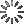• Share

# Recreating Phase Beam in Canvas - Part 2Kushagra Agarwal

### Recreating Android's ICS Phase Beam Live Wallpaper Using Canvas

This is the second part (or the last) of the series that I started a while ago. In this, I will show you how to make the circles blurry and add glowing lines like in the original wallpaper, you might want to take a look on it. I assume that you already completed the first tutorial and experimented with different parameters to get a better understanding of how `canvas` works. Let's recall what we learn't in the previous one:

• Creating a `canvas` and initializing it's context using Javascript.
• Setting the `canvas` `height` and `width` to the window's `height` and `width`.
• Creating gradients and adding one to the `canvas`.
• Creating and animating circles with varying properties using `Math.random()` function.

Now we'll start by first making the circles blurry and mix with the background.

### Step 1 - Making the circles blurry

The best way to make those circles blurry is to create a new radial gradient having three color stops. The first and second color stops will going to be of the same color while the third one is going to be transparent which can be done by using `rgba` colors.

Let's start by creating a new variable and call it blur_grad and then use the `createRadialGradient(start x, start y, initial size, end x, end y, end size)` function on the context `ctx` we created in last tutorial. Now to add the color stops, we'll use the same technique that we used when we created linear gradient.

```var blur_grad = ctx.createRadialGradient(c.x, c.y, 0, c.x, c.y, c.r);
```

You must note that I changed the overall colors of the wallpaper so if you are using the colors from the previous tutorial, then use the same here

Now we just need to change the `ctx.fillStyle = grad` to `ctx.fillStyle = blur_grad` and that will make the circles blurry just like in the original phase beam live wallpaper. You can play with different values to get more interesting results!

### Step 2 - Creating the lines and adding them to canvas

This is pretty same as creating circles. The only difference is that the drawing of the lines is different from that of lines. In case of circles, we used the `ctx.arc()` function while for lines, we'll use a combination of two functions to draw it which are `moveTo()` and `lineTo()`. So for now, just add the function for creating lines just like we did for circles. The code will look like the one below.

```var lines = [];
var lines_count = 15;

for(var i = 0; i < lines_count; i++){
lines.push(new create_lines());
}

function create_lines() {
//Random Positions
this.x = Math.random()*W;
this.y = Math.random()*H;

//Random Velocities
this.vx = 0.1+Math.random()*0.8;
this.vy = -this.vx;

//Random Length
this.l = 5 + Math.random()*20;
}
```

Now to add the lines to canvas, we are going to add another loop in the `draw()` function which will look exactly same as the one we created for circles. The only difference will be the way by which the lines are drawn. To draw a line, we first add our pointer to a random place in canvas by using the `moveTo()` function, and then start drawing from that point to a new point which will be equal to the random length of the line by using `lineTo()` function. You'll understand that by looking at the following code.

```ctx.moveTo(l.x, l.y);
ctx.lineTo(l.x + l.l, l.y - l.l);
ctx.strokeStyle = "rgba(0,120,1,0.5)";
ctx.stroke();
```

You can try again playing with different values, even random things to get some better understanding on how this actually works. Everything else will be same.

### Step 3 - Making the lines glow

Here, instead of using a gradient to make the lines glow, we'll use the shadow functions of canvas. The shadow can be created to any element using two objects namely `shadowColor` and `shadowBlur`. Also, to prevent the circles from getting these shadows or glow, we'll need to save the canvas state before applying the glow to lines and then restore it after applying the glow. To accomplish this, we can use the `save()` and `restore()` functions. These are really very handy when you just want to style some selected elements.

```ctx.save();
ctx.moveTo(l.x, l.y);
ctx.lineTo(l.x + l.l, l.y - l.l);
ctx.strokeStyle = "rgba(0,120,1,0.5)";
ctx.stroke();
ctx.restore();
```

So now, I think the canvas will be looking pretty sweet and just like the Phase Beam wallpaper (not exactly same though). Now take a look at the code by using the tabs above to understand it more. I have also changed the colors to make it look a little bit different but you can always go with the previous one.

If you find anything difficult, or if anything needs more explanation, then drop a comment and I'll help you. Stay tuned from more canvas fun ;)

### Validations

(Errors from your JS or Pre-Processors code)

HTML
CSS
JavaScript

CSSDeck G+